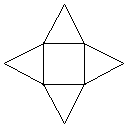In this example, we are going to create a four star with inner square shape in C++ using OpenGL libraries within Microsoft Visual Studio. Following is the problem:This is what we have to create.

``````/*
@Program - Four star with inner square shape using OpenGL, C++
@Author - Amol Nirmala Waman
@Date - 20130716
@Info - IGNOU MCSL-054 part 2 practical exam
@Libraries: opengl32.lib, glu32.lib, glut32.lib
*/``````

## Dependencies

First we have to include dependencies. Namely `windows.h`, `gl.h`, `glu.h`, `glut.h` header files. If your IDE don’t have OpenGL utility library and/or glut toolkit, you can download it. Exam lab does has those.

``````#include<windows.h>
#include<gl/gl.h>
#include<gl/glu.h>  // OpenGL Utility Library
#include<gl/glut.h> // OpenGL Utility Toolkit``````

## Initialize

Now lets initialize the program to define background+foreground color, points, projection etc.

``````void shapeInit (void)
{
glClearColor(0.1, 0.1, 0.1, 0.1); // Background color = White
glColor3f(0.0, 0.0, 0.0); // RGB foreground color = Black
glPointSize(1.0); // Default 1.0
glMatrixMode(GL_PROJECTION);
gluOrtho2D(0.0, 800.0, 0.0, 600.0);
}``````

## Draw the shape

Time to draw the shape! First we will build the inner square starting from its left vertical line. See how vertex changes while we draw the lines.

Once the inner square is in place, start drawing the triangles. Left triangle -> Right triangle -> Top triangle -> Bottom triangle is a good order to draw triangles. So that there will be less chance to mess with any line.

``````void shapeDisplay (void)
{
glClear(GL_COLOR_BUFFER_BIT);
glBegin(GL_LINES); // GL_LINES, GL_POLYGON etc

/* 1. inner square */
// left vertical line
glVertex2i(200, 200); // xy points
glVertex2i(200, 400);
// right vertical line
glVertex2i(400, 200);
glVertex2i(400, 400);
// bot horizontal line
glVertex2i(200, 200);
glVertex2i(400, 200);
// top horizontal line
glVertex2i(200, 400);
glVertex2i(400, 400);

/* 2. left triangle */
// bot cross line
glVertex2i(000, 300);
glVertex2i(200, 200);
// top cross line
glVertex2i(000, 300);
glVertex2i(200, 400);

/* 3. right triangle */
// bot cross line
glVertex2i(400, 200);
glVertex2i(600, 300);
// top cross line
glVertex2i(400, 400);
glVertex2i(600, 300);

/* 4. top triangle */
// left cross line
glVertex2i(200, 400);
glVertex2i(300, 600);
// right cross line
glVertex2i(400, 400);
glVertex2i(300, 600);

/* 5. bot triangle */
// left cross line
glVertex2i(200, 400);
glVertex2i(300, 000);
// right cross line
glVertex2i(300, 000);
glVertex2i(400, 200);

glEnd();
glFlush();
}``````

## Build into main()

OK, our shape program is now ready to call it in main function. Please check the order of `shapeDisplay()` and `shapeInit()` functions.

``````void main (int argc, char **argv)
{
glutInit(&argc, argv);
glutInitDisplayMode(GLUT_SINGLE|GLUT_RGB);
glutInitWindowSize(400,400);
glutInitWindowPosition(100,100);
glutCreateWindow("MCSL-054 OpenGL");
glutDisplayFunc(shapeDisplay);
shapeInit();
glutMainLoop();
}``````

We are done! Now run your program to see it on the screen!

## Output:Output